# I/Q Modulation

NI RF Vector Signal Analyzers (NI-RFSA 18.1) Help

Edition Date: June 2018

Part Number: 372058U-01

View Product InfoNI-RFSA 18.1 HelpNI-RFSA 18.2.1 HelpNI-RFSA 19.1 Help

I/Q is a common way to represent message signals in modern communication systems. As described in the Modulation topic, there are two fundamental ways (degrees of freedom) to produce a modulated wave from a carrier wave. You can either perturb its amplitude or its phase. I/Q signaling allows you to simultaneously change both amplitude and phase. Using both degrees of freedom when modulating a carrier allows greater spectral efficiency with regard to bits per hertz of channel bandwidth (for a given bits per second transmission rate) than if using only one degree of freedom.

To simultaneously perturb both the amplitude and phase of a carrier, two canonical forms of frequency-translating transmitters simultaneously use the two degrees of freedom in modulation. These canonical forms are described in the following sections.

## Canonical Polar ModulationIn polar modulation, the message signal is split into two components: R(t) controls the carrier wave amplitude changes, and φ(t) drives the carrier wave phase changes.

## Canonical Rectangular Modulation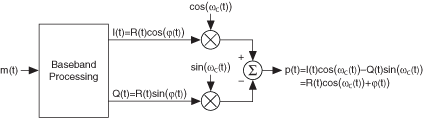In rectangular modulation, the message signal is also split into two components: I(t) controls the in-phase carrier wave changes, and Q(t) controls the quadrature-phase carrier wave changes.

## The Mathematical Relationship

The modulated outputs of the two canonical forms are mathematically and physically equivalent. To show their equality, you can compare and translate between the polar and rectangular representations.

Begin with the equation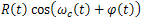.

Plug in the following trigonometric identity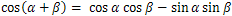to yield the following equation: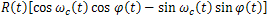.

When you simplify this equation, you have the following relationship: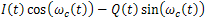where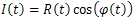ωc = 2fc

fc is the carrier frequency in hertz.

The rectangular form of modulation, often called I/Q Modulation, has become popular for certain technical reasons.

In the rectangular modulation figure, the real baseband signals of I(t) and Q(t) are created (in some way) to contain all the information of message input m(t). (The polar R(t) and φ(t) also contain the information of m(t).) Because the carriers cos(ωc(t)) and sin(ωc(t)) are orthogonal functions, we use the terminology of quadrature modulation, where the signal applied to the cosine mixer is called the In-phase component (I), and the signal applied to the sine mixer is call the Quadrature-phase component (Q). The I and Q designations are useful because the I(t) baseband signal is applied to the cosine mixer, and the Q(t) baseband signal is applied to the sine mixer.

The analytical relationship between the polar form (R(t) and φ(t)) of the baseband signal and Cartesian form (I(t) and Q(t)) of the baseband signal is shown in the following figure.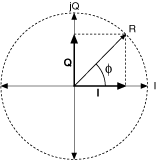The diagram is a "snapshot in time" of the complex (analytical) baseband envelope g(t). Because the diagram is for an arbitrary instant in time, the independent time variable t is dropped. The amplitude of Q is projected onto the imaginary (j) axis and the amplitude of I is projected onto the real axis.Note  For a more thorough analysis of the complex mathematical relationship between the polar and rectangular forms, refer to Digital and Analog Communication Systems by Leon W. Couch.

An I/Q receiver (by definition) recovers a signal containing both amplitude and phase modulation. A block diagram of an I/Q receiver follows.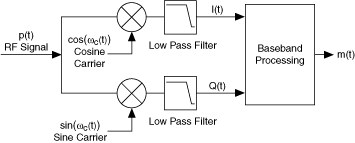For the incoming signal, p(t), the normalized outputs of the cosine and sine mixers are respectively: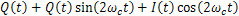After the mixers, the lowpass filters remove all the high-frequency 2ωct terms, leaving us the recovered I(t) and Q(t) signals.

The diagrams and discussion in this section are ideal—they imply that the original message signal m(t) is recovered along the transmitter-path-receiver chain. This implication is useful when focusing purely upon the signaling method. In reality, however, the receiver returns a version of m(t) corrupted by noise and distortion impairments.

The PXI vector signal analyzer measures the unit under test (UUT) noise and distortion impairments and determines if the performance of the UUT is sufficient for the desired transmission scheme. Because the I/Q signal representation is relatively straightforward (and also has practical and physical application in communication systems), it is conceptually used to evaluate the noise and distortion performance of these communication systems.

Modulation